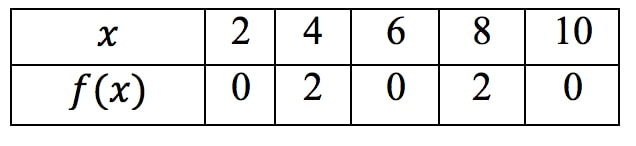Estimating derivatives from a table - Derivatives

Estimating derivatives from a table

Lessons

Use the table below to estimate the following derivatives as accurately as possible: